• 整个SMO算法包含两个部分：求解两个变量二次规划的解析方法和选择变量的启发式算法。 两个变量的二次规划求解方法 假设 α 1 , α 2 \alpha_1, \alpha_2 α 1 ​ , α 2 ​ 为变量，其余的量为固定量 设问题的...
原问题：
$\min_\alpha\frac{1}{2}\sum_{i=1}^N\sum_{j=1}^N\alpha_i\alpha_jy_iy_jK(x_i,x_j)-\sum_{i=1}^N\alpha_i$
s.t.
$\sum_{i=1}^N\alpha_iy_i=0$
$0\leqslant\alpha_i\leqslant C, i=1,2,\cdots,N$
SMO算法的基本思想是：
如果所有变量的解都满足最优化问题的KKT条件，那么这个最优化问题的解就得到了。否则，选择两个变量，固定其他变量，针对这两个变量构建一个二次规划问题。
这样做的目的是，通过求解两个变量的二次规划问题，能不断靠近原有凸二次规划问题的解，并且计算方法有解析方法。
子问题有两个变量，其中一个为违反KKT条件最严重的那一个，另一个由约束条件自动确定。由与约束条件的存在，子问题实际上只有一个自由变量。
整个SMO算法包含两个部分：求解两个变量二次规划的解析方法和选择变量的启发式算法。
两个变量的二次规划求解方法
假设$\alpha_1, \alpha_2$为变量，其余的量为固定量
设问题的原始可行解为$\alpha_1^{old}, \alpha_2^{old}$,最优解为$\alpha_1^{new},\alpha_2^{new}$，并且在沿着约束方向未经剪辑时$\alpha_2$的最优解为$\alpha_2^{new,unc}$
由于约束条件存在，因此$\alpha_2^{new}$的取值范围为：
$L\leqslant\alpha_2^{new}\leqslant H$
其中$L$与$H$是$\alpha_2^{new}$所在的对角线段端点的界。
如果$y_1\not=y_2$，则
$L=\max(0,\alpha_2^{old}-\alpha_1^{old}),\,\,\,\,\,H=\min(C,C+\alpha_2^{old}-\alpha_1^{old})$
如果$y_1=y_2$，则
$L=\max(0,\alpha_2^{old}+\alpha_1^{old}-C),\,\,\,\,\,H=\min(C,\alpha_2^{old}+\alpha_1^{old})$
下面先求未经剪辑的最优解$\alpha_2^{new,unc}$，记
$g(x)=\sum_{i=1}^N\alpha_iy_iK(x_i,x)+b$
令
$E_i=g(x_i)-y_i=(\sum_{j=1}^N\alpha_jy_jK(x_j,x_i)+b), i=1,2$
$当i=1,2时，E_i为函数g(x)对输入x_i的预测值与真实输出y_i之差。$
定理：
最优化问题沿着约束方向未经剪辑的解时
$\alpha_2^{new,unc}=\alpha_2^{old}+\frac{y_2(E_1-E_2)}{\eta}$
其中，
$\eta=K_{11}+K_{22}-K_{12}=||\phi(x_1)-\phi(x_2)||^2$
其中，$\phi(x)$是输入空间到特征空间的映射
经剪辑后$\alpha_2$的解，$\alpha_2^{new}=$
$H\,\,\,\,\,\,\,,\alpha_2^{new,unc}>H$
$\alpha_2^{new,unc}\,\,\,\,\,\,\,,L\leqslant\alpha_2^{new,unc}\leqslant H$
$L\,\,\,\,\,\,\,,\alpha_2^{new,unc}
再由$\alpha_2^{new}$求得$\alpha_1^{new}$:
$\alpha_1^{new}=\alpha_1^{old}+y_1y_2(\alpha_2^{old}-\alpha_2^{new})$
变量的选择方法
SMO算法在每个子问题中需要选择两个变量进行优化，并且至少一个变量是违反KKT条件的。
第一个变量的选择
外层循环，挑选在训练样本中选取违反KKT条件最严重的样本点，并将其对应的变量作为第一个变量。检验训练样本点$(x_i,x_j)$是否满足KKT条件，即
$\alpha_i=0\Leftrightarrow y_ig(x_i)\geqslant1$
$0<\alpha_i
$\alpha_i=C\Leftrightarrow y_ig(x_i)\leqslant1$
其中，$g(x_i)=\sum_{j=1}^N\alpha_jy_jK(x_i,x_j)+b$
该检验是在$\epsilon$范围内进行的。在检验过程中，外层循环首先遍历所有满足条件$0<\alpha_i的样本点，如果都满足，则遍历整个数据集。
第二个变量选择
内层循环，假设已经寻找到了第一个变量$\alpha_1$，现在需要寻找$\alpha_2$，选择标准是希望能使$\alpha_2$有足够大的变化。
思路1：由于$\alpha_2^{new}$是依赖于$|E_1-E_2|$的，，我们只需选择$\alpha_2$，使其对应的$|E_1-E_2|$最大。由于$E_1$已经确定，若$E_1$是正的，就选择最小的$E_i$作为$E_2$，如果$E_1$是负的，就选择最大的$E_i$作为$E_2$。
思路2：若思路1所选的$\alpha_2$不能使目标函数有足够的下降，则遍历所有在间隔边界上的支持向量点，依次作为$\alpha_2$试用，直到目标函数有足够的下降；若还是找不到，则遍历整个数据集；如何还是找不到，则抛弃$\alpha_1$，重新寻找新的$\alpha_1$。
计算阈值$b$与差值$E_i$
每次完成两个变量的优化后，都需要重新计算阈值$b$，当$0<\alpha_1^{new}时，由KKT条件可知：
$\sum_{i=1}^N\alpha_iy_iK_{i1}+b=y_1$
于是
$b_1^{new}=y_i-\sum_{i=3}^N\alpha_iy_iK_{i1}-\alpha_1^{new}y_1K_{11}-\alpha_2^{new}y_2K_{21}$
用$E_1,E_2$进行表示，有
$b_1^{new}=-E_1-y_1K_{11}(\alpha_1^{new}-\alpha_1^{old})-y_2K_{21}(\alpha_2^{new}-\alpha_2^{old})+b^{old}$
同理，如果$0<\alpha_2^{new}，那么
$b_2^{new}=-E_2-y_1K_{12}(\alpha_1^{new}-\alpha_1^{old})-y_2K_{22}(\alpha_2^{new}-\alpha_2^{old})+b^{old}$
如果$b_1^{new},b_2^{new}$同时满足条件$0<\alpha_i^{new}，那么$b_1^{new}=b_2^{new}$；如果$b_1^{new},b_2^{new}$是0或者C，那么$b_1^{new},b_2^{new}$以及他们之间的数都是符合KKT条件的阈值，选择他们的中点作为$b^{new}$。
在每次完成两个变量的优化后，还必须更新对应的$E_i$值
$E_i^{new}=\sum_Sy_j\alpha_iK(x_i,x_j)+b^{new}-y_i$
其中，S是所有支持向量$x_j$的集合。
代码如下：
from sklearn import datasets
import matplotlib.pyplot as plt # 画图工具
import numpy as np

X, y = datasets.make_classification(n_samples=100, n_features=2, n_informative=2, n_redundant=0, n_repeated=0, n_classes=2, n_clusters_per_class=1, random_state=2)
for i in range(X.shape):
if y[i] == 0:
y[i] = -1
#plt.scatter(X[:, 0], X[:, 1], c=y)
# plt.show()

class SMO:
def __init__(self, X, y, C, toler, maxIter):  # samples labels constance tolerate maxIteration
self.X = X
self.y = y
self.C = C
self.toler = toler
self.maxIter = maxIter
self.N = self.X.shape
self.b = 0
self.a = np.zeros(self.N)
self.K = np.zeros((self.N, self.N))
self.o = 0.5
for i in range(self.N):
for j in range(self.N):
t = 0
for k in range(self.X.shape):
t += (self.X[i][k] - self.X[j][k])**2
self.K[i][j] = np.exp(-t/(2*self.o**2))

def select_J(self, i):  # random choose j which is not equal to i.
j = i
while j == i:
j = np.random.randint(0, self.N)
return j

def fix_alpha(self, a, H, L):
if a > H:
a = H
if a < L:
a = L
return a

def cal_Ei(self, j):
t = 0
for i in range(self.N):
t += self.a[i]*self.y[i]*self.K[i][j]
return t + self.b - self.y[j]

def update(self):
iter = 0
while iter < self.maxIter:
alphaPairsChanged = 0
for i in range(self.N):
Ei = self.cal_Ei(i)
if (Ei < -self.toler and self.a[i] < self.C) or (Ei > self.toler and self.a[i] > 0):
j = self.select_J(i)  # choose j != i
Ej = self.cal_Ei(j)
aiold = self.a[i]
ajold = self.a[j]
if self.y[i] != self.y[j]:
L = max(0, self.a[j] - self.a[i])
H = min(self.C, self.C + self.a[j] - self.a[i])
else:
L = max(0, self.a[j] + self.a[i] - self.C)
H = min(self.C, self.a[j] + self.a[i])
if L == H:
print("L==H")
continue
eta = self.K[i][i] + self.K[j][j] - 2*self.K[i][j]
if eta <= 0 :
print('eta <= 0')
continue
ajnew = ajold + self.y[j]*(Ei - Ej)/eta
ajnew = self.fix_alpha(ajnew, H, L)
if abs(ajnew - ajold) < 0.00001:
print("j not moving enough")
continue
self.a[j] = self.fix_alpha(ajnew, H, L)
self.a[i] = aiold + self.y[i]*self.y[j]*(ajold - ajnew)
b1 = -Ei - self.y[i]*self.K[i][i]*(self.a[i] - aiold) - self.y[j]*self.K[i][j]*(ajnew - ajold) + self.b
b2 = -Ej - self.y[i]*self.K[i][j]*(self.a[i] - aiold) - self.y[j]*self.K[j][j]*(ajnew - ajold) + self.b
if 0 < self.a[i] < self.C:
self.b = b1
elif 0 < self.a[j] < self.C:
self.b = b2
else:
self.b = (b1 + b2)/2
alphaPairsChanged += 1
if alphaPairsChanged == 0:
iter += 1
else:
iter = 0
return self.b, self.a

def score(self, X, y):
K = np.zeros((X.shape, self.N))
for i in range(X.shape):
for j in range(self.N):
t = 0
for k in range(self.X.shape):
t += (self.X[i][k] - self.X[j][k]) ** 2
K[i][j] = np.exp(-t / (2 * self.o ** 2))
y_pre = []
for i in range(X.shape):
t = 0
for j in range(self.N):
t += self.a[j]*self.y[j]*K[i][j]
y_pre.append(t + self.b)
s = 0
for i in range(X.shape):
if y_pre[i] < 0 and y[i] == -1:
s += 1
elif y_pre[i] > 0 and y[i] == 1:
s += 1
return s/X.shape

L = SMO(X, y, 1, 0.001, 100)
b, a = L.update()
score = L.score(X, y)
print('b = {}'.format(b))
print('a = {}'.format(a))
print('the accuracy of this SVM is {}'.format(score))

运行代码，所得到的结果为：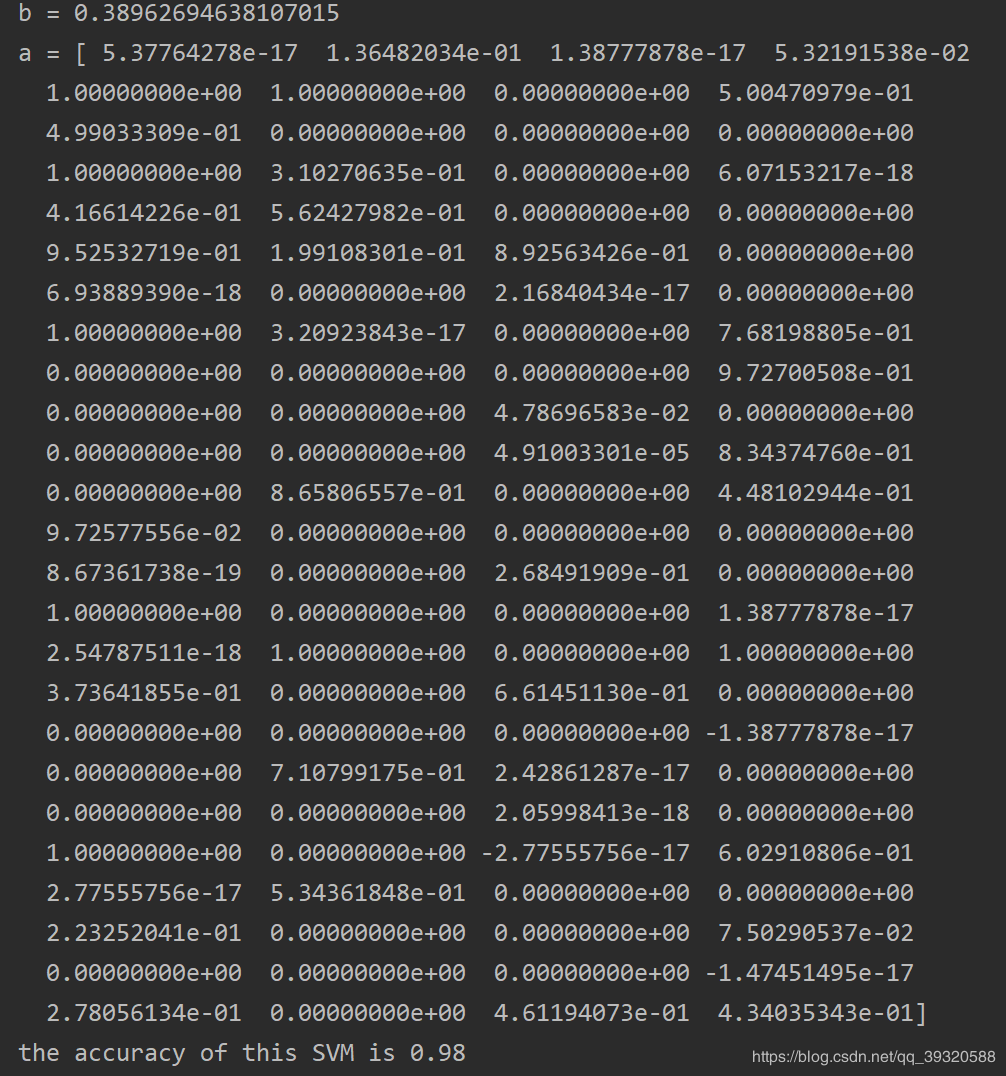分别是对偶问题当中的变量$b,\alpha$
以及最终训练出的SVM模型的精确度为98%


展开全文机器学习 svm
• python算法动态规划-高抛低吸-完全背包问题 一、 关键： **1、完全背包。**把今天与明天各物品的价格差作为物品的价值，物品的原本价格看作物品的体积，转化为了完全背包问题，一共有n天，做n - 1完全背包问题...
python写算法动态规划-高抛低吸-完全背包问题
一、关键：
1、完全背包。
把今天与明天各物品的价格差作为物品的价值，物品的原本价格看作物品的体积，转化为了完全背包问题，一共有n天，做n - 1次完全背包问题即可。
！！一点细节，第二天大于第一天才规划，省时间。
2、转化的关键。
首先很重要的一点就是，物品可以当日购买，当日出售。所以：可以把第一天购买第四天才卖的商品看作第二天出售再购买，第三天出售再购买直到第四天出售不购买，赚的钱是一样多的。
！！所以基于上面的思路，不需要考虑隔天的销售情况，我们只需要考虑第二天的售买情况即可。
3、贪心。
完全背包可以让每天都赚到最大，那么到第n天的一定是最多的。
二、题目如下：
纪念品，预测，洛谷p5662
三、代码
1、怎么说呢，我尽力了，也超时了。。。。。t,n,m=[int(s) for s in input().split()]
val=[[int(s) for s in input().split()]for j in range(t)]
val.append(*n)

for i in range(t):
dp=*(m+1)#t-1个背包,最后一天一定不算
for j in range(n):
if val[i+1][j]>val[i][j]:#第二天更大，算
for k in range(val[i][j],m+1):
dp[k]=max(dp[k],dp[k-val[i][j]]+val[i+1][j]-val[i][j])
m+=dp[m]
print(m)

2、用c++吧。
#include <iostream>
#include <cstring>

using namespace std;

const int N = 105, M = 10005;

int w[N][N];
int f[M];
int t, n, m;

int main()
{
scanf("%d%d%d", &t, &n, &m);

for (int i = 1; i <= t; i ++ )
for (int j = 1; j <= n; j ++ )
scanf("%d", &w[i][j]);

for (int i = 1; i < t; i ++ )
{
memset(f, 0, sizeof f);//每次做dp前归零数组

for (int j = 1; j <= n; j ++ )
if (w[i + 1][j] > w[i][j])  //优化
for (int k = w[i][j]; k <= m; k ++ )
f[k] = max(f[k], f[k - w[i][j]] + w[i + 1][j] - w[i][j]);//优化后的完全背包模板

m += f[m];
}

printf("%d\n", m);

return 0;
}



展开全文• 下面我们就来拿二次方程练练手，在python中求取二次方程。 示例：计算二次方程式 ax**2 + bx + c = 0 注意：首先要导入 math模块 代码： import math import unicodedata def is_number(s): try: float(s) ...
• 5 Python基本算法5.1递归5.1.1基本情况的分析5.1.2动态规划5.2搜索和排序5.2.1无序表搜索方式5.2.2有序表的搜索5.2.3哈希表级目录级目录 5.1递归 递归算法遵守三个原则，①所有的递归算法都有基本情况；②递归...
5 Python基本算法（ing）5.1递归5.1.1基本情况的分析5.1.2动态规划5.2搜索和排序5.2.1无序表搜索方式5.2.2有序表的搜索5.2.3哈希表
5.1递归
递归算法遵守三个原则，①所有的递归算法都有基本情况；②递归算法必须改变它本身的状态并且向基本情况靠近；③递归算法必须递归地调用自身。
5.1.1基本情况的分析
对比下面的两个将十进制100转化成二进制的程序，在分析的时候，可以把基本情况就是任何的余数都需要再进行一次二进制的转换，所以就嵌套调用了tostr()函数。
from pythonds import Stack
def change(s,t):
dig = "0123456789ABCDEF"
st = Stack()
rea = ""
while s > 0:
m = s%t
st.push(m)
s = s//t
while not st.isEmpty():
rea = rea + dig[st.pop()]
return rea
print(chang(100,2))

from pythonds import Stack
rstack = Stack()
rea = ""
def tostr(n,base):
covstr = "0123456789ABCDEF"
if n < base:
rstack.push(covstr[n])
else:
rstack.push(covstr[n % base])
tostr(n // base,base)
tostr(8,2)
while not rstack.isEmpty():
rea = rea + rstack.pop()
print(rea)

5.1.2动态规划
动态规划在计算机中，是为了求解决策过程最优化的过程。以找零时使用最少个数的零钱来举例，零钱有100、50、20、10、5和1块的面值，假设拿10块钱买了3块钱的肥仔水，找零时当然是一张5块两张1块的零钱个数最少。我们在分析时，应该先从要找零的范围内最大面值的开始，然后看第二大面值的…依次查询。这种方法也叫“贪婪算法”，也就是动态规划的一种情况。
5.2搜索和排序
5.2.1无序表搜索方式
无序表只能通过依次的顺序搜索。
def sequentialSearch(alist, item):
pos = 0
found = False
while pos < len(alist) and not found:
if alist[pos] == item:
found = True
else:
pos = pos+1
return found

无序表的搜索时间复杂度是O(n)，因为目标在表内时，最好情况是1次就查到，最坏是n次才查到，平均是n/2，目标不在表内时，无论好坏都是查n
次，平均也就是n次。
5.2.2有序表的搜索
顺序搜索：
def orderedSequentialSearch(alist, item):
pos = 0
found = False
stop = False
while pos < len(alist) and not found and not stop:
if alist[pos] == item:
found = True
else:
if alist[pos] > item:
stop = True
else:
pos = pos+1
return found

二分法搜索：
def binarySearch(alist, item):
if len(alist) == 0:
return False
else:
midpoint = len(alist)//2
if alist[midpoint]==item:
return True
else:
if item<alist[midpoint]:
return binarySearch(alist[:midpoint],item)
else:
return binarySearch(alist[midpoint+1:],item)


5.2.3哈希表
哈希表也叫散列表，其中的每个存放元素位置称为槽，哈希表利用哈希函数来将表中的元素和所属位置对应起来。哈希表为了方便存储，通常都是利用字典来定义数据的，这样可以通过键来替代找值。
哈希表的哈希函数很多时候在运算规则上会导致不同的数据要存放到同一个槽里，为了避免这种冲突，


展开全文数据库 机器学习
• 本学期上了最优化课程，学习...这里基于均值-方差分析方法，针对以确定收益水平下的最小化风险为目标的投资组合问题建立了具有等式约束的凸二次规划模型，并分别利用GUROBI求解器和一种高阶牛顿算法对其进行求解。 ...
本学期上了最优化课程，学习到了一些凸优化的知识，想要尝试将这些优化理论应用到实际中。

这里基于均值-方差分析方法，针对以确定收益水平下的最小化风险为目标的投资组合问题建立了具有等式约束的凸二次规划模型，并分别利用GUROBI求解器和一种高阶牛顿算法对其进行求解。

模型描述如下（公式太多的地方就直接贴图片了）：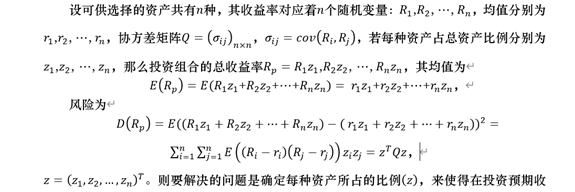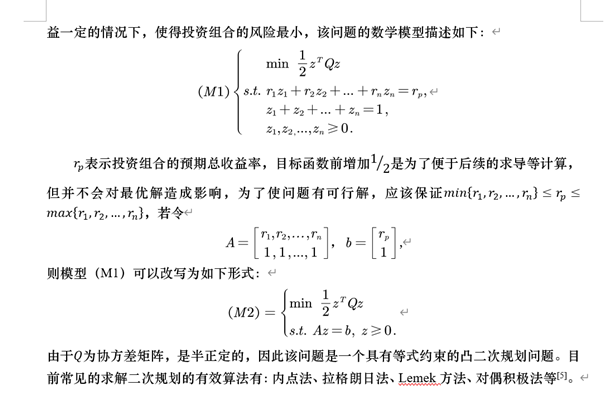分别利用Gurobi求解器和高阶牛顿法求解：

Gurobi：Gurobi是美国Gurobi Optimization公司开发的大规模优化求解器，目前已经成为综合能力全球领先的数学规划求解器，作为一个全局优化器，Gurobi支持的模型类型包括：连续和混合整数线性问题、凸目标或约束连续和混合整数二次问题、含有指对数、三角函数、高阶多项式目标和约束以及任何形式的分段约束的非线性问题等。另外，Gurobi提供了方便轻巧的API接口，支持C++、Java、Python等多种语言，同时支持包括Windows、Linux以及OS在内的多种平台。

基于绝对值光华逼近的高阶牛顿法（公式真的多我是真的懒，依旧贴图片）：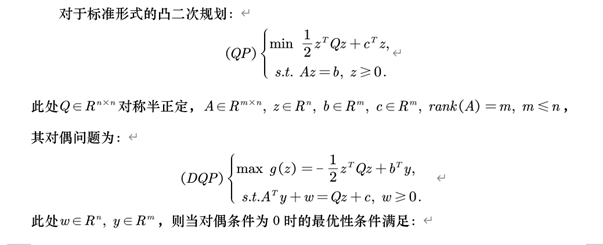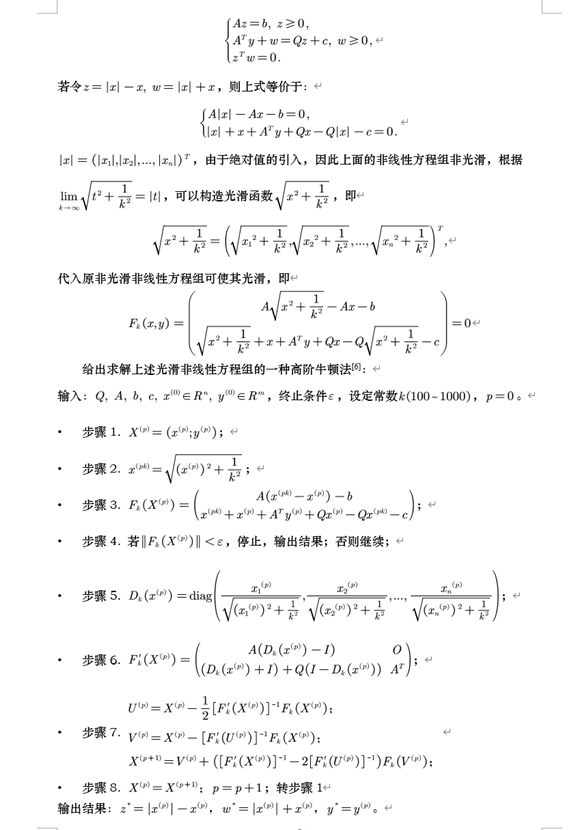代码如下：

from gurobipy import GRB, GurobiError
import gurobipy as gp
from numpy import *
import math
import pandas as pd

c=array([,,])
Q=array([[0.2,0.3,-0.01],[0.3,2.4,0.5],[-0.01,0.5,1.1]])
A=array([[0.06,0.12,0.09],[1,1,1]])
b=array([[0.06],])
c=array([,,])
x=array([,,])
y=array([,])
k=1000
e=0.000001

def guroby_solver(Q,A,b,c):
try:

m = gp.Model("QP_guroby")
var = []
constr = 0
obj = 0

for i in range(len(A)):
var.append(m.addVar(lb=0, vtype=GRB.CONTINUOUS, name='var[%d]' % i))  #定义变量

for i in range(len(A)):
constr = 0
for j in range(len(A)):
if A[i][j] != 0:
constr = constr + A[i][j] * var[j]
m.addConstr(constr == b[i], name='constraint %d' % i)     #添加约束

for i in range(len(A)):
for j in range(len(A)):
obj = obj + Q[i][j] * var[i] * var[j]
for i in range(len(A)):
obj = obj + c[i] * var[i]
m.setObjective(obj, GRB.MINIMIZE)    #添加目标函数

m.optimize()
variable=[]
for v in m.getVars():
print(v.varName, v.x)
variable.append(v.x)
print('Obj:', m.objVal)

except GurobiError:
print('Error reported')
return variable,m.objVal          #GUROBI求解器求解函数

def newton(x,y,e,k,Q,A,b,c):

def cal_pk(m):
x_pk=zeros((len(m),1))
for i in range(len(m)):
x_pk[i]=math.sqrt(m[i]**2+1/(k**2))
return x_pk

def cal_Fk(X,m):
x_split=X[:m,:]
y_split=X[m:,:]
Fk_up=dot(A,cal_pk(x_split)-x_split)-b
Fk_down=cal_pk(x_split)+x_split+ \
dot(A.T,y_split)+dot(Q,x_split)-dot(Q,cal_pk(x_split))-c
Fk=vstack((Fk_up,Fk_down))
return Fk

def cal_Fk_grad(X,m,n):
x_split=X[:m,:]
Dk=zeros((m,m))
for i in range(m):
Dk[i][i]=x_split[i]/math.sqrt(x_split[i]**2+1/(k**2))
O=zeros((n,n))
Fk_grad_up=hstack((dot(A,Dk-identity(3)),O))
Fk_grad_down=hstack((Dk+identity(3)+dot(Q,identity(3)-Dk),A.T))
Fk_grad=vstack((Fk_grad_up,Fk_grad_down))
return Fk_grad

X=vstack((x,y))
while True:
if dot(cal_Fk(X,len(x)).T,cal_Fk(X,len(x)))<e:
break
U=X-0.5*dot(linalg.inv(cal_Fk_grad(X,len(x),len(y))),cal_Fk(X,len(x)))
V=X-dot(linalg.inv(cal_Fk_grad(U,len(x),len(y))),cal_Fk(X,len(x)))
X=V+dot(linalg.inv(cal_Fk_grad(X,len(x),len(y))) \
-2*linalg.inv(cal_Fk_grad(U,len(x),len(y))),cal_Fk(V,len(x)))
re_x=X[:len(x),:]
z=zeros((len(x),1))
for i in range(len(x)):
z[i]=abs(re_x[i])-re_x[i]
mid=dot(Q,z)
obj_fuc=dot(z.T,mid)+dot(c.T,z)
print('高阶牛顿法的最优解为：',z)
print('目标函数值为：',obj_fuc)

return z,obj_fuc              #牛顿法求解函数

df=pd.DataFrame(index=range(13),columns=['rp','z1_g','z2_g', \
'z3_g','obj_g','z1_n', \
'z2_n','z3_n','obj_n'])
for i in range(13):
b=0.06+i*0.005
m,n=guroby_solver(Q,A,b,c)
p,q=newton(x,y,e,k,Q,A,b,c)
df['rp'][i]=b
df['z1_g'][i]=m
df['z2_g'][i]=m
df['z3_g'][i]=m
df['obj_g'][i]=n
df['z1_n'][i]=p
df['z2_n'][i]=p
df['z3_n'][i]=p
df['obj_n'][i]=q
df.to_csv('Result.csv')     #将计算结果导入excel

参考文献：

雍龙泉,贾伟,黎延海.基于光滑逼近函数的高阶牛顿法求解凸二次规划[J].科学技术与工程,2021,21(06):2151-2156.


展开全文• 本文实现python版本的有效集算法，有效集...# 有效集算法实现，解决二次规划问题 # min 1/2x.THx+c.Tx # s.t. Ax>=b import numpy as np class Active_set(object): def __init__(self, H, c, A, b): self.H...
• 题目导览丑数斐波那契数列卡特兰数贝尔数项式系数排列系数 丑数 丑数为仅有 2,3或5 的质因子的数。数列1, 2, 3, 4, 5, 6, 8, 9, 10, 12, 15, … 展示了前11个丑数。按照惯例，其中包括1。给定一个数字n，请你找出...
• SMO算法的原理在之前的文章：支持向量机（SVM）凸二次规划的求解——序列最小最优化算法（SMO）原理及python实现有十分详细的介绍，这里直接展示代码 from sklearn import datasets import matplotlib.pyplot as plt...机器学习 svm
• SVM使用SMO算法来解决其中涉及到的二次规划问题。一个简单版本的SMO算法的实现如下： ''' 随机选择随机数，不等于J ''' def selectJrand(i,m): j=i #we want to select any J not equal to i while (j==i): j = ...SVM 迭代
• SVM--简介 支持向量机（Support Vector Machines）是一种二分类模型，它的目的是寻找一个超平面来对样本进行分割，分割的原则是间隔最大化，最终转化为一个凸二次规划问题来求解。 在机器学习领域，是一个有监督的...
• 1、最优化与线性规划 最优化问题的三要素是决策变量、目标函数和约束条件。...但是，由一般线性规划问题所衍生的整数规划、混合规划、0/1规划、二次规划、非线性规划、组合优化问题，则并不是调用某个库函数都能处理.编程语言 人工智能 数学建模
• 目录题目描述解题思路动态规划代码实现Tips 题目描述 给定一个整数数组，其中第 iii 个元素代表了第 iii 天的股票价格 。​ 设计一个算法计算出最大利润。在满足以下约束条件下，你可以尽可能地完成更多的交易（多...leetcode
• 通俗来讲，它是一种二类分类模型，其基本模型定义为特征空间上的间隔最大的线性分类器，其学习策略便是间隔最大化，最终可转化为一个凸二次规划问题的求解。 本文详述SVM的理论基础，并通过Python实现了该算法人工智能 svm 机器学习
• 序列二次规划（SQP）是解决约束优化问题中较好的一种算法，其流程为 scipy.optimize.minimize(fun, x0, args=(), method=None, jac=None, hess=None, hessp=None, bounds=None, constraints=(), tol=None, callback...优化
• 不过，这篇文章对于学习 Python 的小伙伴或者学习 TSP 遗传算法的哥们都有作用。如果需要 Matlab 代码，可以翻阅我其他文章。 如果没有参加过深圳杯或者没选 C 题，那么很好，我这里说一下问题： 题目给出了城市坐标...C
• 如果采用分治法求解，有些子问题被重复计算多，造成计算资源的浪费。 如果能够保存已解决的子问题的答案，而在需要时再找出已求得的答案，就可以避免重复计算，从而得到多项式的时间算法。 （）多项式基本步骤 ...
• 方法：动态规划 这一问题可以转换为求A走了偶数后到达楼顶的情况数。 因为假设A走了2xxx后到达楼顶，则可以视作A走了xxx的同时B也走了剩余的xxx，这时他们必定会相遇。 故可以用动态规划求解，dp[j]表示当A...dfs 数据结构 leetcode
• 支持向量机是由Vapnik等...学习策略是间隔最大化，可形式化求解一个凸二次规划的问题。 1 线性可分支持向量机 给定一个线性可分的训练集，找到一个间隔最大的分隔超平面，将两类数据正确划分。 1.1 函数间隔...
• 1．支持向量机最简单的情况...可以表示为凸二次规划问题，其原始最优化问题为 min⁡w,b12∥w∥2\min _{w, b} \frac{1}{2}\|w\|^{2}w,bmin​21​∥w∥2s.t.yi(w⋅xi+b)−1⩾0,i=1,2,⋯&ThinSpace;,Ns.t. \quad y...SVM
• 动态规划难不倒我一：股票（一交易）：：子数组的最大累加和：三：连续子数组的最大和：四：求路径：五：换钱的最少货币数： 一：股票（一交易）： 假设你有一个数组，其中第 i 个元素是股票在第i 天的价格。 ...
• 在满足以下约束条件下，你可以尽可能地完成更多的交易（多买卖一支股票）: 你不能同时参与多笔交易（你必须在再次购买前出售掉之前的股票）。 卖出股票后，你无法在第天买入股票 (即冷冻期为 1 天)。 我们...leetcode
• SVM python代码实现 支持向量机（support vector machines, SVM）是一种二分类模型，它的基本模型是定义在特征空间上的间隔最大的线性分类器，间隔最大使它有别于...SVM的的学习算法就是求解凸二次规划的最优化算法
• sklearn.svm.SVC 参数说明 经常用到sklearn中的SVC函数，这里把文档中的参数翻译了一些...（PS: libsvm中的二次规划问题的解决算法是SMO）。 sklearn.svm.SVC(C=1.0,kernel='rbf', degree=3, gamma='auto',coef0...svm sklearn svm.SVC SVM参数说明
•编程语言
• 在投资组合优化问题的背景下，这种方法显然比通用二次规划算法更合适。 本文的主要目标是通过以科学语言提供一个有据可查的、分步开源的 CLA 实现来填补文献中的空白。 代码作为 Python 类对象实现，这使得它可以像...
• SMO算法，是求解SVM对偶问题（凸二次规划问题）的一种算法，由Platt在1998提出。下面会基于python实现SMO算法。但传统的SVM只能实现2类划分，因此下面会基于one vs one 思想处理多类划分问题。* one vs one* 其做法...机器学习# python二次规划算法python 订阅